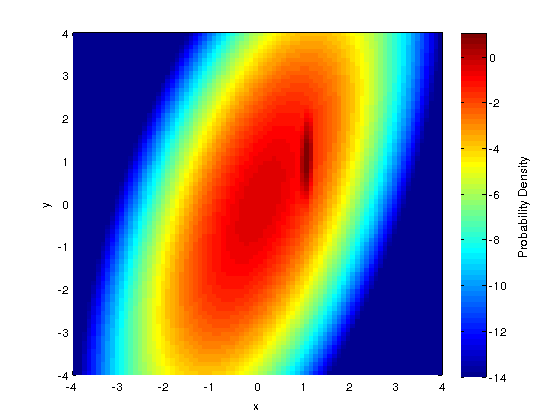## Monday, 6 May 2013

### Logarithmic Density Plots MATLAB or Octave: Two Gaussians Different Scales

Common visualization of 3D data manifest as surface and mesh plots. Data may contain values which are different in several orders of magnitude if not more. In this post, I will demonstrate how to transform your 3D data values to logarithmic scale so that this magnitude discrepancy is not washed out due to linear scaling. I will give code sample using MATLAB but example should be applicable to Octave as well.
But the procedure should be generic and easily be written in any other language of your choice.

The most common 3D data set appear as values over spatial dimensions. For example third dimension could be a probability distribution. Let's say we use two multivariate gaussians with different means and covariances using mvnpdf.  In the first figure we see only a small region; visiable for us. However there are data points which are washed out due to different scale. In the code below we re-write the data's zeros to a very small value (the matrix F), say 1-e14, so that we can convert the values to logarithmic scale,  remember that log(0) is an -Inf and not plotable. Resulting figure gives us more information about our data.

  1 2 3 4 5 6 7 8 9 10 11 12 13 14 15 16 17 18 19 20 21 22 23 24 25 26 27 28 29 30 31 32 33 34 35 36 37 % Two Gaussians on the plane mu1 = [0 0]; sigma1 = [.25 .3; .3 1]; mu2 = [1, 1]; sigma2 = [.0025 .003; .003 0.1]; x = -4:.1:4; y = -4:.1:4; [X, Y] = meshgrid(x,y); F = mvnpdf([X(:) Y(:)], mu1, sigma1) + mvnpdf([X(:) Y(:)], mu2, sigma2); F = reshape(F,length(x),length(y)); figure(1) pcolor(X, Y, F); shading flat xlabel('x'); ylabel('y'); c=colorbar; ylabel(c,'Probability Density') ; figure(2) % Fill zeros with small number smallN = 1e-14; [~,y] = size(F); getZeroIds = @(colN) find(F(:,colN) < smallN); applyAllCols = @(ii) evalin('base', ['colN=', sprintf('%d',ii) ,';F(getZeroIds(colN), colN)=smallN;']); arrayfun(applyAllCols, 1:y); % pcolor(X, Y, log10(F)); shading flat xlabel('x'); ylabel('y'); c=colorbar; ylabel(c,'Probability Density') ; % clear all close all [x y] = meshgrid(-5:0.5:5, -5:0.5:5); z = - (1 - x.^2 -y.^2) .* exp(-(x.^2 + y.^2)).* exp(-(0.5*x.^2 - y.^2)); surf(z);Figure 1: Two Gaussians in linear scale.Figure 2: Two Gaussians in logarithmic scale (see the  code above)